# Tag: Manin

Last time I mentioned the talk “From noncommutative geometry to the tropical geometry of the scaling site” by Alain Connes, culminating in the canonical isomorphism (last slide of the talk)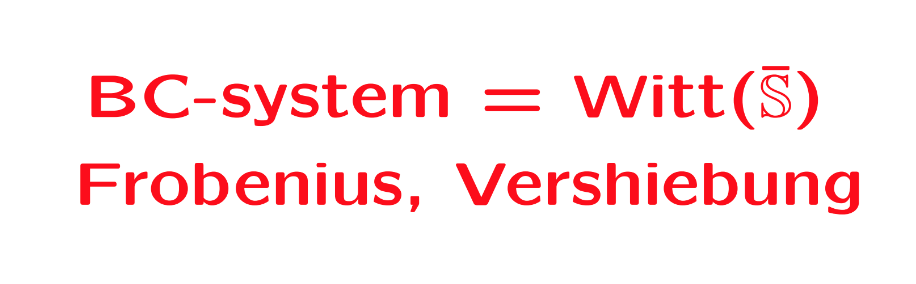Or rather, what is actually proved in his paper with Caterina Consani BC-system, absolute cyclotomy and the quantized calculus (and which they conjectured previously to be the case in Segal’s Gamma rings and universal arithmetic), is a canonical isomorphism between the $\lambda$-rings
$\mathbb{Z}[\mathbb{Q}/\mathbb{Z}] \simeq \mathbb{W}_0(\overline{\mathbb{S}})$
The left hand side is the integral groupring of the additive quotient-group $\mathbb{Q}/\mathbb{Z}$, or if you prefer, $\mathbb{Z}[\mathbf{\mu}_{\infty}]$ the integral groupring of the multiplicative group of all roots of unity $\mathbf{\mu}_{\infty}$.

The power maps on $\mathbf{\mu}_{\infty}$ equip $\mathbb{Z}[\mathbf{\mu}_{\infty}]$ with a $\lambda$-ring structure, that is, a family of commuting endomorphisms $\sigma_n$ with $\sigma_n(\zeta) = \zeta^n$ for all $\zeta \in \mathbf{\mu}_{\infty}$, and a family of linear maps $\rho_n$ induced by requiring for all $\zeta \in \mathbf{\mu}_{\infty}$ that
$\rho_n(\zeta) = \sum_{\mu^n=\zeta} \mu$
The maps $\sigma_n$ and $\rho_n$ are used to construct an integral version of the Bost-Connes algebra describing the Bost-Connes sytem, a quantum statistical dynamical system.

On the right hand side, $\mathbb{S}$ is the sphere spectrum (an object from stable homotopy theory) and $\overline{\mathbb{S}}$ its ‘algebraic closure’, that is, adding all abstract roots of unity.

The ring $\mathbb{W}_0(\overline{\mathbb{S}})$ is a generalisation to the world of spectra of the Almkvist-ring $\mathbb{W}_0(R)$ defined for any commutative ring $R$, constructed from pairs $(E,f)$ where $E$ is a projective $R$-module of finite rank and $f$ an $R$-endomorphism on it. Addition and multiplication are coming from direct sums and tensor products of such pairs, with zero element the pair $(0,0)$ and unit element the pair $(R,1_R)$. The ring $\mathbb{W}_0(R)$ is then the quotient-ring obtained by dividing out the ideal consisting of all zero-pairs $(E,0)$.

The ring $\mathbb{W}_0(R)$ becomes a $\lambda$-ring via the Frobenius endomorphisms $F_n$ sending a pair $(E,f)$ to the pair $(E,f^n)$, and we also have a collection of linear maps on $\mathbb{W}_0(R)$, the ‘Verschiebung’-maps which send a pair $(E,f)$ to the pair $(E^{\oplus n},F)$ with
$F = \begin{bmatrix} 0 & 0 & 0 & \cdots & f \\ 1 & 0 & 0 & \cdots & 0 \\ 0 & 1 & 0 & \cdots & 0 \\ \vdots & \vdots & \vdots & & \vdots \\ 0 & 0 & 0 & \cdots & 1 \end{bmatrix}$
Connes and Consani define a notion of modules and their endomorphisms for $\mathbb{S}$ and $\overline{\mathbb{S}}$, allowing them to define in a similar way the rings $\mathbb{W}_0(\mathbb{S})$ and $\mathbb{W}_0(\overline{\mathbb{S}})$, with corresponding maps $F_n$ and $V_n$. They then establish an isomorphism with $\mathbb{Z}[\mathbb{Q}/\mathbb{Z}]$ such that the maps $(F_n,V_n)$ correspond to $(\sigma_n,\rho_n)$.

But, do we really have the go to spectra to achieve this?

All this reminds me of an old idea of Yuri Manin mentioned in the introduction of his paper Cyclotomy and analytic geometry over $\mathbb{F}_1$, and later elaborated in section two of his paper with Matilde Marcolli Homotopy types and geometries below $\mathbf{Spec}(\mathbb{Z})$.

Take a manifold $M$ with a diffeomorphism $f$ and consider the corresponding discrete dynamical system by iterating the diffeomorphism. In such situations it is important to investigate the periodic orbits, or the fix-points $Fix(M,f^n)$ for all $n$. If we are in a situation that the number of fixed points is finite we can package these numbers in the Artin-Mazur zeta function
$\zeta_{AM}(M,f) = exp(\sum_{n=1}^{\infty} \frac{\# Fix(M,f^n)}{n}t^n)$
and investigate the properties of this function.

To connect this type of problem to Almkvist-like rings, Manin considers the Morse-Smale dynamical systems, a structural stable diffeomorphism $f$, having a finite number of non-wandering points on a compact manifold $M$.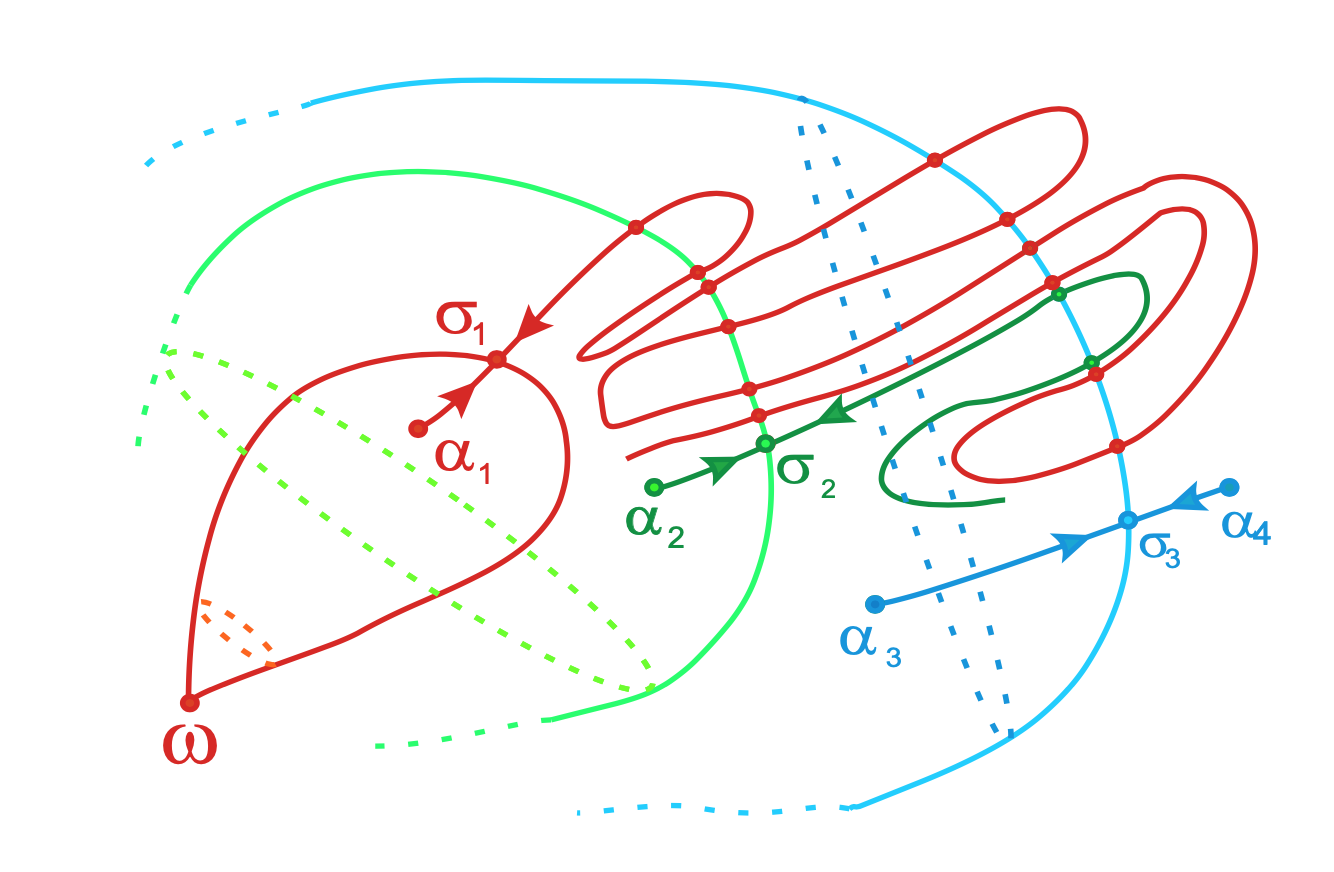From Topological classification of Morse-Smale diffeomorphisms on 3-manifolds

In such a situation $f_{\ast}$ acts on homology $H_k(M,\mathbb{Z})$, which are free $\mathbb{Z}$-modules of finite rank, as a matrix $M_f$ having only roots of unity as its eigenvalues.

Manin argues that this action is similar to the action of the Frobenius on etale cohomology groups, in which case the eigenvalues are Weil numbers. That is, one might view roots of unity as Weil numbers in characteristic one.

Clearly, all relevant data $(H_k(M,\mathbb{Z}),f_{\ast})$ belongs to the $\lambda$-subring of $\mathbb{W}_0(\mathbb{Z})$ generated by all pairs $(E,f)$ such that $M_f$ is diagonalisable and all its eigenvalues are either $0$ or roots of unity.

If we denote for any ring $R$ by $\mathbb{W}_1(R)$ this $\lambda$-subring of $\mathbb{W}_0(R)$, probably one would obtain canonical isomorphisms

– between $\mathbb{W}_1(\mathbb{Z})$ and the invariant part of the integral groupring $\mathbb{Z}[\mathbb{Q}/\mathbb{Z}]$ for the action of the group $Aut(\mathbb{Q}/\mathbb{Z}) = \widehat{\mathbb{Z}}^*$, and

– between $\mathbb{Z}[\mathbb{Q}/\mathbb{Z}]$ and $\mathbb{W}_1(\mathbb{Z}(\mathbf{\mu}_{\infty}))$ where $\mathbb{Z}(\mathbf{\mu}_{\infty})$ is the ring obtained by adjoining to $\mathbb{Z}$ all roots of unity.

A Belyi-extender (or dessinflateur) is a rational function $q(t) = \frac{f(t)}{g(t)} \in \mathbb{Q}(t)$ that defines a map
$q : \mathbb{P}^1_{\mathbb{C}} \rightarrow \mathbb{P}^1_{\mathbb{C}}$
unramified outside $\{ 0,1,\infty \}$, and has the property that $q(\{ 0,1,\infty \}) \subseteq \{ 0,1,\infty \}$.

An example of such a Belyi-extender is the power map $q(t)=t^n$, which is totally ramified in $0$ and $\infty$ and we clearly have that $q(0)=0,~q(1)=1$ and $q(\infty)=\infty$.

The composition of two Belyi-extenders is again an extender, and we get a rather mysterious monoid $\mathcal{E}$ of all Belyi-extenders.

Very little seems to be known about this monoid. Its units form the symmetric group $S_3$ which is the automrphism group of $\mathbb{P}^1_{\mathbb{C}} – \{ 0,1,\infty \}$, and mapping an extender $q$ to its degree gives a monoid map $\mathcal{E} \rightarrow \mathbb{N}_+^{\times}$ to the multiplicative monoid of positive natural numbers.

If one relaxes the condition of $q(t) \in \mathbb{Q}(t)$ to being defined over its algebraic closure $\overline{\mathbb{Q}}$, then such maps/functions have been known for some time under the name of dynamical Belyi-functions, for example in Zvonkin’s Belyi Functions: Examples, Properties, and Applications (section 6).

Here, one is interested in the complex dynamical system of iterations of $q$, that is, the limit-behaviour of the orbits
$\{ z,q(z),q^2(z),q^3(z),… \}$
for all complex numbers $z \in \mathbb{C}$.

In general, the 2-sphere $\mathbb{P}^1_{\mathbb{C}} = S^2$ has a finite number of open sets (the Fatou domains) where the limit behaviour of the series is similar, and the union of these open sets is dense in $S^2$. The complement of the Fatou domains is the Julia set of the function, of which we might expect a nice fractal picture.

Let’s take again the power map $q(t)=t^n$. For a complex number $z$ lying outside the unit disc, the series $\{ z,z^n,z^{2n},… \}$ has limit point $\infty$ and for those lying inside the unit circle, this limit is $0$. So, here we have two Fatou domains (interior and exterior of the unit circle) and the Julia set of the power map is the (boring?) unit circle.

Fortunately, there are indeed dynamical Belyi-maps having a more pleasant looking Julia set, such as this one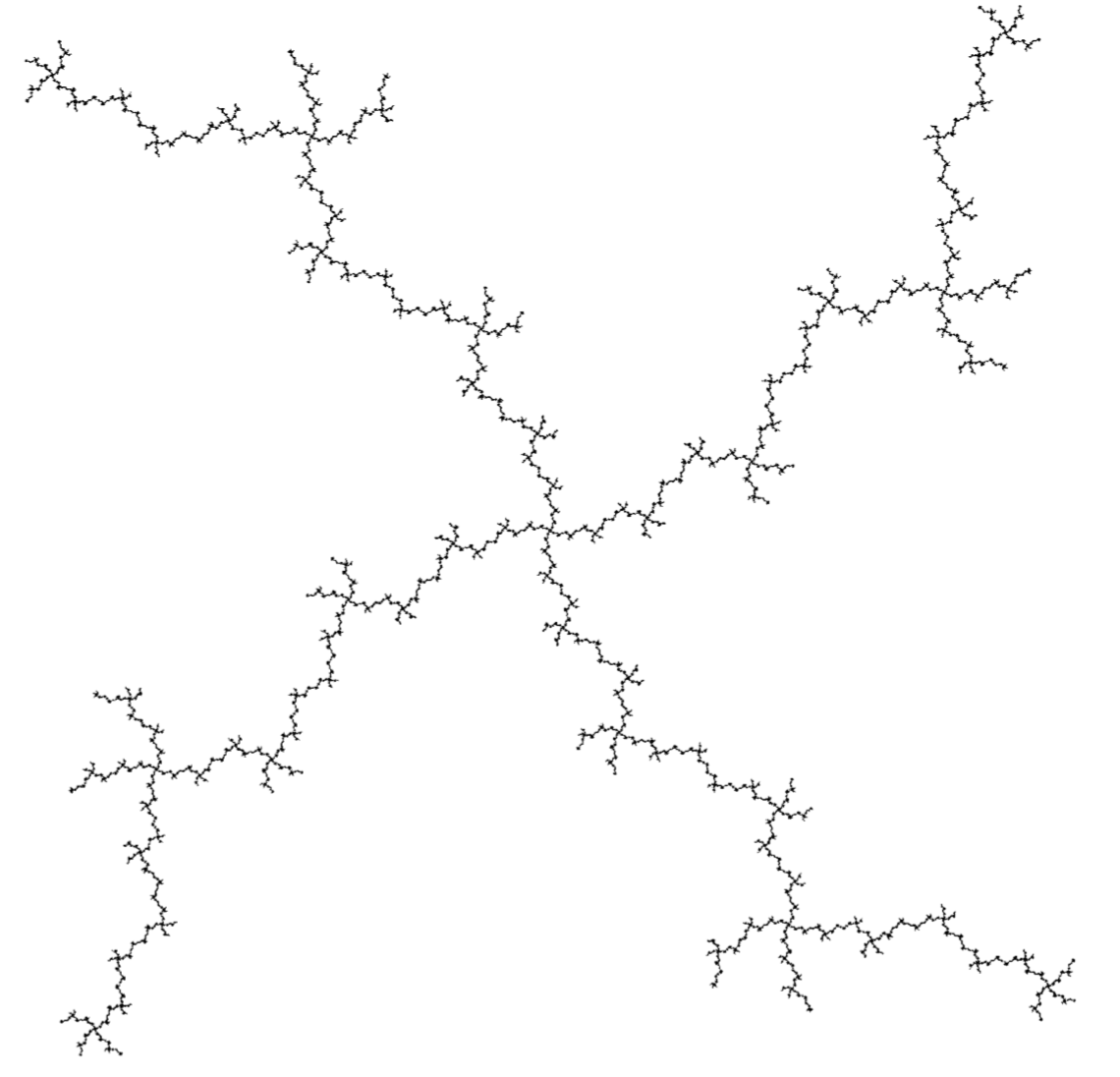But then, many dynamical Belyi-maps (and Belyi-extenders) are systems of an entirely different nature, they are completely chaotic, meaning that their Julia set is the whole $2$-sphere! Nowhere do we find an open region where points share the same limit behaviour… (the butterfly effect).

There’s a nice sufficient condition for chaotic behaviour, due to Dennis Sullivan, which is pretty easy to check for dynamical Belyi-maps.

A periodic point for $q(t)$ is a point $p \in S^2 = \mathbb{P}^1_{\mathbb{C}}$ such that $p = q^m(p)$ for some $m > 1$. A critical point is one such that either $q(p) = \infty$ or $q'(p)=0$.

Sullivan’s result is that $q(t)$ is completely chaotic when all its critical points $p$ become eventually periodic, that is some $q^k(p)$ is periodic, but $p$ itself is not periodic.

For a Belyi-map $q(t)$ the critical points are either comlex numbers mapping to $\infty$ or the inverse images of $0$ or $1$ (that is, the black or white dots in the dessin of $q(t)$) which are not leaf-vertices of the dessin.

Let’s do an example, already used by Sullivan himself:
$q(t) = (\frac{t-2}{t})^2$
This is a Belyi-function, and in fact a Belyi-extender as it is defined over $\mathbb{Q}$ and we have that $q(0)=\infty$, $q(1)=1$ and $q(\infty)=1$. The corresponding dessin is (inverse images of $\infty$ are marked with an $\ast$)The critical points $0$ and $2$ are not periodic, but they become eventually periodic:

$2 \rightarrow^q 0 \rightarrow^q \infty \rightarrow^q 1 \rightarrow^q 1$
and $1$ is periodic.

For a general Belyi-extender $q$, we have that the image under $q$ of any critical point is among $\{ 0,1,\infty \}$ and because we demand that $q(\{ 0,1,\infty \}) \subseteq \{ 0,1,\infty \}$, every critical point of $q$ eventually becomes periodic.

If we want to avoid the corresponding dynamical system to be completely chaotic, we have to ensure that one of the periodic points among $\{ 0,1,\infty \}$ (and there is at least one of those) must be critical.

Let’s consider the very special Belyi-extenders $q$ having the additional property that $q(0)=0$, $q(1)=1$ and $q(\infty)=\infty$, then all three of them are periodic.

So, the system is always completely chaotic unless the black dot at $0$ is not a leaf-vertex of the dessin, or the white dot at $1$ is not a leaf-vertex, or the degree of the region determined by the starred $\infty$ is at least two.

Going back to the mystery Manin-Marcolli sub-monoid of $\mathcal{E}$, it might explain why it is a good idea to restrict to very special Belyi-extenders having associated dessin a $2$-coloured tree, for then the periodic point $\infty$ is critical (the degree of the outside region is at least two), and therefore the conditions of Sullivan’s theorem are not satisfied. So, these Belyi-extenders do not necessarily have to be completely chaotic. (tbc)

A Belyi-extender (or dessinflateur) $\beta$ of degree $d$ is a quotient of two polynomials with rational coefficients
$\beta(t) = \frac{f(t)}{g(t)}$
with the special properties that for each complex number $c$ the polynomial equation of degree $d$ in $t$
$f(t)-c g(t)=0$
has $d$ distinct solutions, except perhaps for $c=0$ or $c=1$, and, in addition, we have that
$\beta(0),\beta(1),\beta(\infty) \in \{ 0,1,\infty \}$

Let’s take for instance the power maps $\beta_n(t)=t^n$.

For every $c$ the degree $n$ polynomial $t^n – c = 0$ has exactly $n$ distinct solutions, except for $c=0$, when there is just one. And, clearly we have that $0^n=0$, $1^n=1$ and $\infty^n=\infty$. So, $\beta_n$ is a Belyi-extender of degree $n$.

A cute observation being that if $\beta$ is a Belyi-extender of degree $d$, and $\beta’$ is an extender of degree $d’$, then $\beta \circ \beta’$ is again a Belyi-extender, this time of degree $d.d’$.

That is, Belyi-extenders form a monoid under composition!

In our example, $\beta_n \circ \beta_m = \beta_{n.m}$. So, the power-maps are a sub-monoid of the Belyi-extenders, isomorphic to the multiplicative monoid $\mathbb{N}_{\times}$ of strictly positive natural numbers.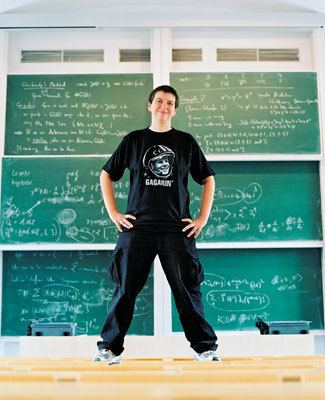In their paper Quantum statistical mechanics of the absolute Galois group, Yuri I. Manin and Matilde Marcolli say they use the full monoid of Belyi-extenders to act on all Grothendieck’s dessins d’enfant.

But, they attach properties to these Belyi-extenders which they don’t have, in general. That’s fine, as they foresee in Remark 2.21 of their paper that the construction works equally well for any suitable sub-monoid, as long as this sub-monoid contains all power-map exenders.

I’m trying to figure out what the maximal mystery sub-monoid of extenders is satisfying all the properties they need for their proofs.

But first, let us see what Belyi-extenders have to do with dessins d’enfant.In his user-friendlier period, Grothendieck told us how to draw a picture, which he called a dessin d’enfant, of an extender $\beta(t) = \frac{f(t)}{g(t)}$ of degree $d$:

Look at all complex solutions of $f(t)=0$ and label them with a black dot (and add a black dot at $\infty$ if $\beta(\infty)=0$). Now, look at all complex solutions of $f(t)-g(t)=0$ and label them with a white dot (and add a white dot at $\infty$ if $\beta(\infty)=1$).

Now comes the fun part.

Because $\beta$ has exactly $d$ pre-images for all real numbers $\lambda$ in the open interval $(0,1)$ (and $\beta$ is continuous), we can connect the black dots with the white dots by $d$ edges (the pre-images of the open interval $(0,1)$), giving us a $2$-coloured graph.

For the power-maps $\beta_n(t)=t^n$, we have just one black dot at $0$ (being the only solution of $t^n=0$), and $n$ white dots at the $n$-th roots of unity (the solutions of $x^n-1=0$). Any $\lambda \in (0,1)$ has as its $n$ pre-images the numbers $\zeta_i.\sqrt[n]{\lambda}$ with $\zeta_i$ an $n$-th root of unity, so we get here as picture an $n$-star. Here for $n=5$: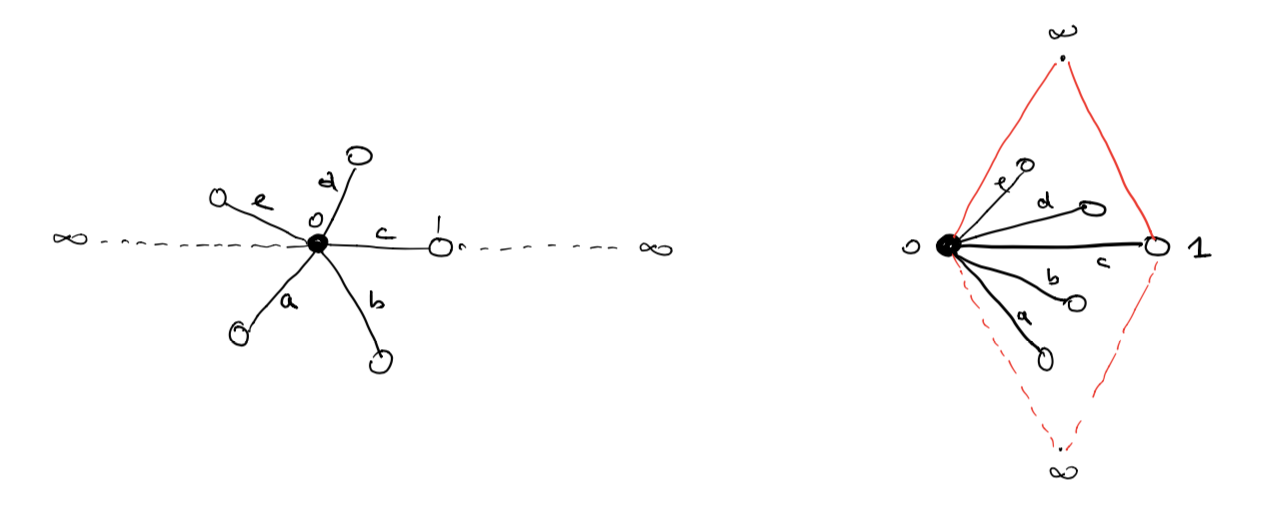This dessin should be viewed on the 2-sphere, with the antipodal point of $0$ being $\infty$, so projecting from $\infty$ gives a homeomorphism between the 2-sphere and $\mathbb{C} \cup \{ \infty \}$.

To get all information of the dessin (including possible dots at infinity) it is best to slice the sphere open along the real segments $(\infty,0)$ and $(1,\infty)$ and flatten it to form a ‘diamond’ with the upper triangle corresponding to the closed upper semisphere and the lower triangle to the open lower semisphere.

In the picture above, the right hand side is the dessin drawn in the diamond, and this representation will be important when we come to the action of extenders on more general Grothendieck dessins d’enfant.

Okay, let’s try to get some information about the monoid $\mathcal{E}$ of all Belyi-extenders.

What are its invertible elements?

Well, we’ve seen that the degree of a composition of two extenders is the product of their degrees, so invertible elements must have degree $1$, so are automorphisms of $\mathbb{P}^1_{\mathbb{C}} – \{ 0,1,\infty \} = S^2-\{ 0,1,\infty \}$ permuting the set $\{ 0,1,\infty \}$.

They form the symmetric group $S_3$ on $3$-letters and correspond to the Belyi-extenders
$t,~1-t,~\frac{1}{t},~\frac{1}{1-t},~\frac{t-1}{t},~\frac{t}{t-1}$
You can compose these units with an extender to get anther extender of the same degree where the roles of $0,1$ and $\infty$ are changed.

For example, if you want to colour all your white dots black and the black dots white, you compose with the unit $1-t$.

Manin and Marcolli use this and claim that you can transform any extender $\eta$ to an extender $\gamma$ by composing with a unit, such that $\gamma(0)=0, \gamma(1)=1$ and $\gamma(\infty)=\infty$.

That’s fine as long as your original extender $\eta$ maps $\{ 0,1,\infty \}$ onto $\{ 0,1,\infty \}$, but usually a Belyi-extender only maps into $\{ 0,1,\infty \}$.

Here are some extenders of degree three (taken from Melanie Wood’s paper Belyi-extending maps and the Galois action on dessins d’enfants):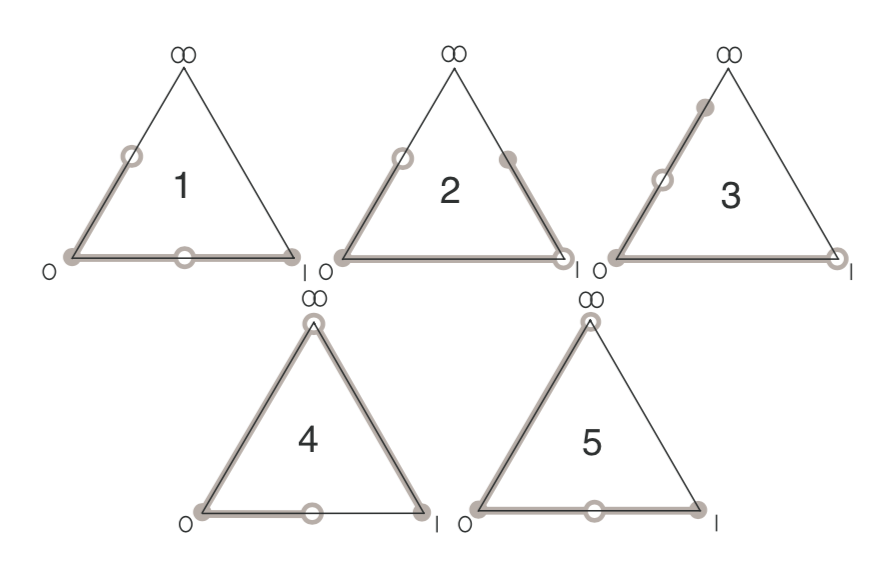with dessin $5$ corresponding to the Belyi-extender
$\beta(t) = \frac{t^2(t-1)}{(t-\frac{4}{3})^3}$
with $\beta(0)=0=\beta(1)$ and $\beta(\infty) = 1$.

So, a first property of the mystery Manin-Marcolli monoid $\mathcal{E}_{MMM}$ must surely be that all its elements $\gamma(t)$ map $\{ 0,1,\infty \}$ onto $\{ 0,1,\infty \}$, for they use this property a number of times, for instance to construct a monoid map
$\mathcal{E}_{MMM} \rightarrow M_2(\mathbb{Z})^+ \qquad \gamma \mapsto \begin{bmatrix} d & m-1 \\ 0 & 1 \end{bmatrix}$
where $d$ is the degree of $\gamma$ and $m$ is the number of black dots in the dessin (or white dots for that matter).

Further, they seem to believe that the dessin of any Belyi-extender must be a 2-coloured tree.

Already last time we’ve encountered a Belyi-extender $\zeta(t) = \frac{27 t^2(t-1)^2}{4(t^2-t+1)^3}$ with dessin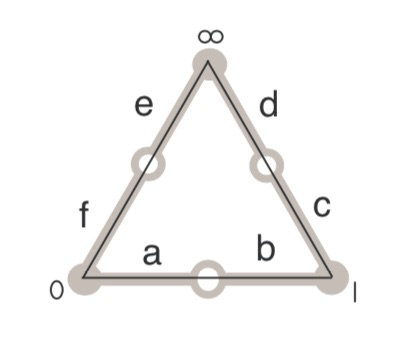But then, you may argue, this extender sends all of $0,1$ and $\infty$ to $0$, so it cannot belong to $\mathcal{E}_{MMM}$.

Here’s a trick to construct Belyi-extenders from Belyi-maps $\beta : \mathbb{P}^1 \rightarrow \mathbb{P}^1$, defined over $\mathbb{Q}$ and having the property that there are rational points in the fibers over $0,1$ and $\infty$.

Let’s take an example, the ‘monstrous dessin’ corresponding to the congruence subgroup $\Gamma_0(2)$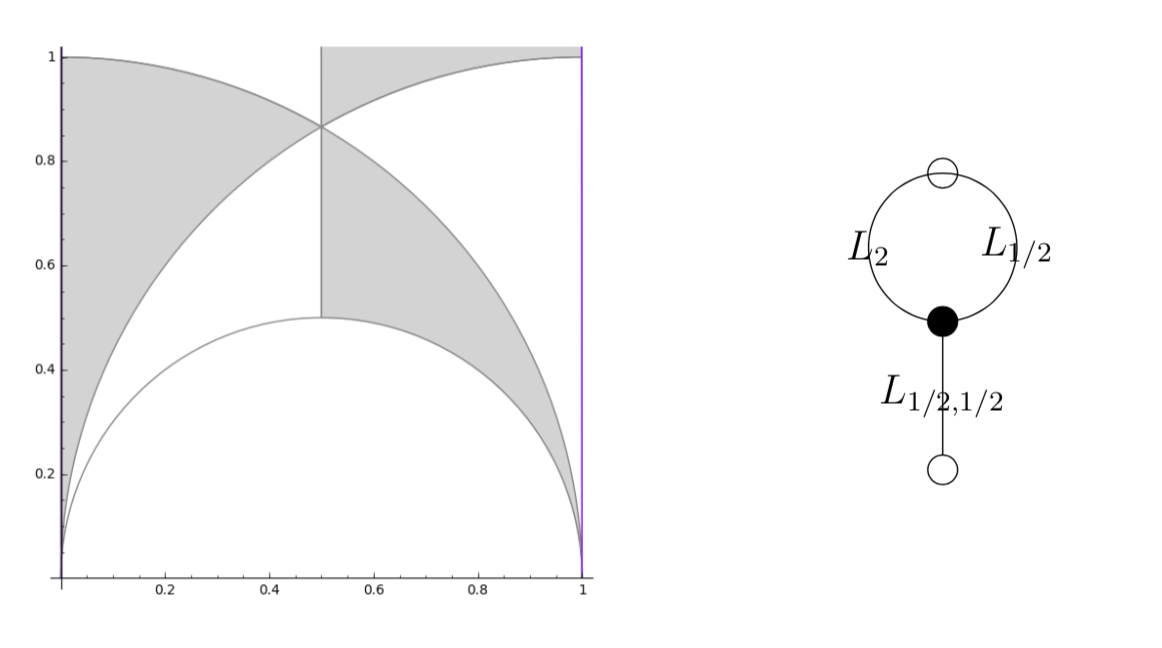with map $\beta(t) = \frac{(t+256)^3}{1728 t^2}$.

As it stands, $\beta$ is not a Belyi-extender because it does not map $1$ into $\{ 0,1,\infty \}$. But we have that
$-256 \in \beta^{-1}(0),~\infty \in \beta^{-1}(\infty),~\text{and}~512,-64 \in \beta^{-1}(1)$
(the last one follows from $(t+256)^2-1728 t^3=(t-512)^2(t+64)$).

We can now pre-compose $\beta$ with the automorphism (defined over $\mathbb{Q}$) sending $0$ to $-256$, $1$ to $-64$ and fixing $\infty$ to get a Belyi-extender
$\gamma(t) = \frac{(192t)^3}{1728(192t-256)^2}$
which maps $\gamma(0)=0,~\gamma(1)=1$ and $\gamma(\infty)=\infty$ (so belongs to $\mathcal{E}_{MMM}$) with the same dessin, which is not a tree,

That is, $\mathcal{E}_{MMM}$ can at best consist only of those Belyi-extenders $\gamma(t)$ that map $\{ 0,1,\infty \}$ onto $\{ 0,1,\infty \}$ and such that their dessin is a tree.

Let me stop, for now, by asking for a reference (or counterexample) to perhaps the most startling claim in the Manin-Marcolli paper, namely that any 2-coloured tree can be realised as the dessin of a Belyi-extender!

I’m trying to get into the latest Manin-Marcolli paper Quantum Statistical Mechanics of the Absolute Galois Group on how to create from Grothendieck’s dessins d’enfant a quantum system, generalising the Bost-Connes system to the non-Abelian part of the absolute Galois group $Gal(\overline{\mathbb{Q}}/\mathbb{Q})$.

In doing so they want to extend the action of the multiplicative monoid $\mathbb{N}_{\times}$ by power maps on the roots of unity to the action of a larger monoid on all dessins d’enfants.

Here they use an idea, originally due to Jordan Ellenberg, worked out by Melanie Wood in her paper Belyi-extending maps and the Galois action on dessins d’enfants.To grasp this, it’s best to remember what dessins have to do with Belyi maps, which are maps defined over $\overline{\mathbb{Q}}$
$\pi : \Sigma \rightarrow \mathbb{P}^1$
from a Riemann surface $\Sigma$ to the complex projective line (aka the 2-sphere), ramified only in $0,1$ and $\infty$. The dessin determining $\pi$ is the 2-coloured graph on the surface $\Sigma$ with as black vertices the pre-images of $0$, white vertices the pre-images of $1$ and these vertices are joined by the lifts of the closed interval $[0,1]$, so the number of edges is equal to the degree $d$ of the map.

Wood considers a very special subclass of these maps, which she calls Belyi-extender maps, of the form
$\gamma : \mathbb{P}^1 \rightarrow \mathbb{P}^1$
defined over $\mathbb{Q}$ with the additional property that $\gamma$ maps $\{ 0,1,\infty \}$ into $\{ 0,1,\infty \}$.

The upshot being that post-compositions of Belyi’s with Belyi-extenders $\gamma \circ \pi$ are again Belyi maps, and if two Belyi’s $\pi$ and $\pi’$ lie in the same Galois orbit, then so must all $\gamma \circ \pi$ and $\gamma \circ \pi’$.

The crucial Ellenberg-Wood idea is then to construct “new Galois invariants” of dessins by checking existing and easily computable Galois invariants on the dessins of the Belyi’s $\gamma \circ \pi$.

For this we need to know how to draw the dessin of $\gamma \circ \pi$ on $\Sigma$ if we know the dessins of $\pi$ and of the Belyi-extender $\gamma$. Here’s the procedure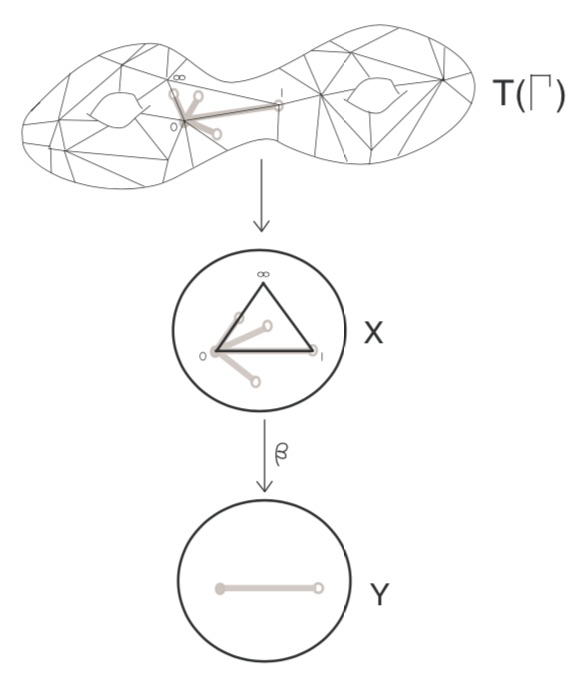Here, the middle dessin is that of the Belyi-extender $\gamma$ (which in this case is the power map $t \rightarrow t^4$) and the upper graph is the unmarked dessin of $\pi$.

One has to replace each of the black-white edges in the dessin of $\pi$ by the dessin of the expander $\gamma$, but one must be very careful in respecting the orientations on the two dessins. In the upper picture just one edge is replaced and one has to do this for all edges in a compatible manner.

Thus, a Belyi-expander $\gamma$ inflates the dessin $\pi$ with factor the degree of $\gamma$. For this reason i prefer to call them dessinflateurs, a contraction of dessin+inflator.

In her paper, Melanie Wood says she can separate dessins for which all known Galois invariants were the same, such as these two dessins,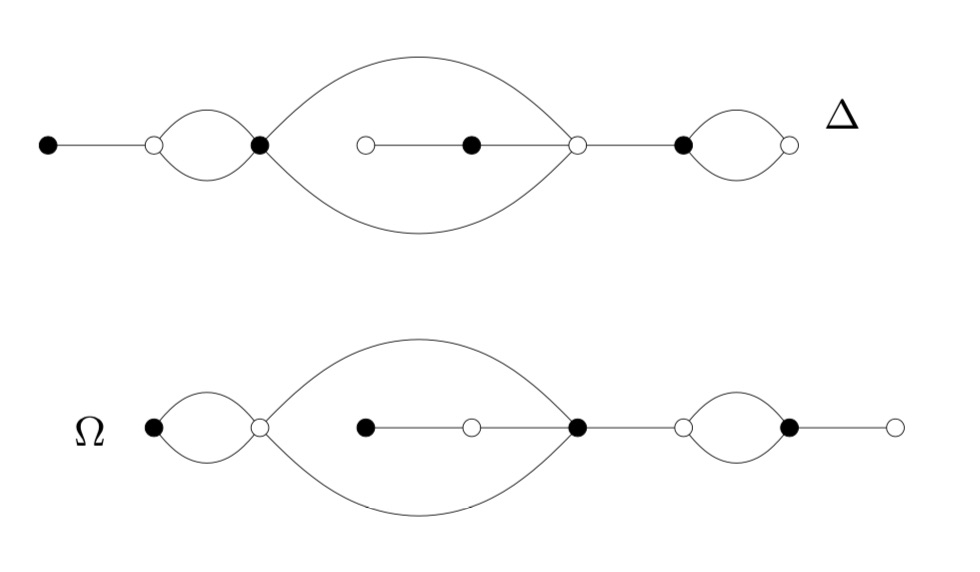by inflating them with a suitable Belyi-extender and computing the monodromy group of the inflated dessin.

This monodromy group is the permutation group generated by two elements, the first one gives the permutation on the edges given by walking counter-clockwise around all black vertices, the second by walking around all white vertices.

For example, by labelling the edges of $\Delta$, its monodromy is generated by the permutations $(2,3,5,4)(1,6)(8,10,9)$ and $(1,3,2)(4,7,5,8)(9,10)$ and GAP tells us that the order of this group is $1814400$. For $\Omega$ the generating permutations are $(1,2)(3,6,4,7)(8,9,10)$ and $(1,2,4,3)(5,6)(7,9,8)$, giving an isomorphic group.

Let’s inflate these dessins using the Belyi-extender $\gamma(t) = -\frac{27}{4}(t^3-t^2)$ with corresponding dessin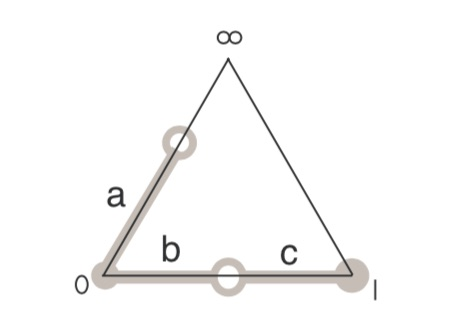It took me a couple of attempts before I got the inflated dessins correct (as i knew from Wood that this simple extender would not separate the dessins). Inflated $\Omega$ on top: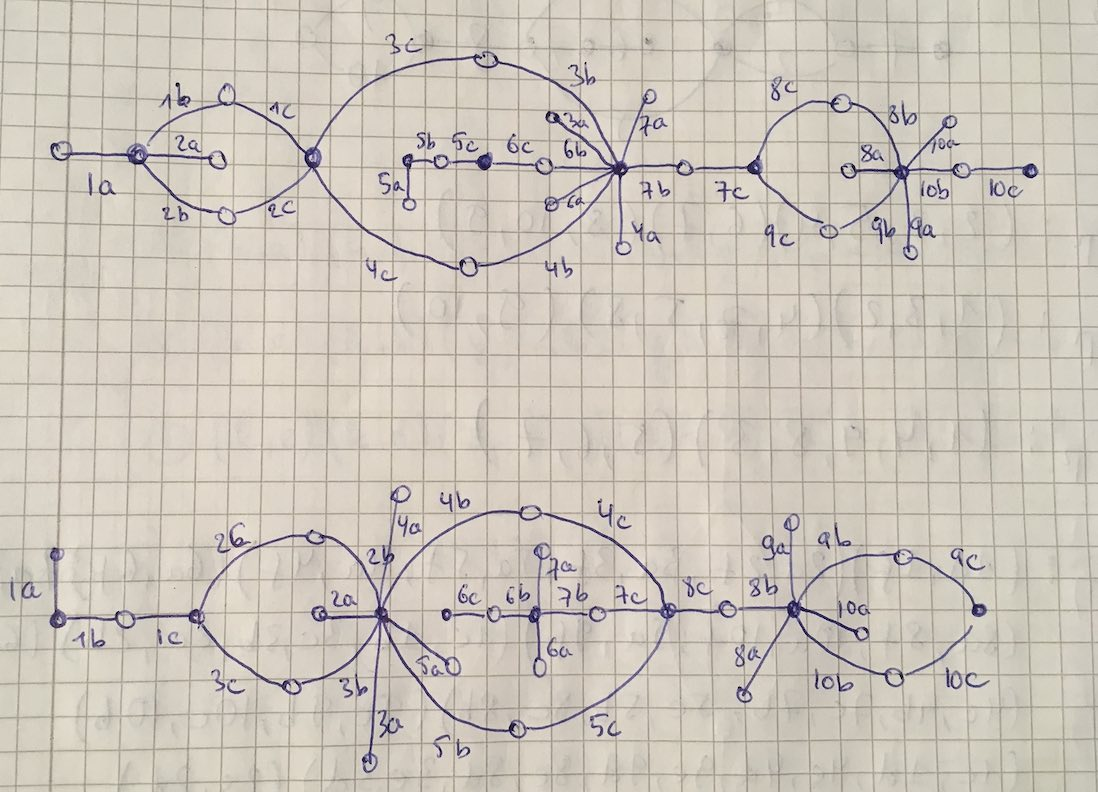Both dessins give a monodromy group of order $35838544379904000000$.

Now we’re ready to do serious work.

Melanie Wood uses in her paper the extender $\zeta(t)=\frac{27 t^2(t-1)^2}{4(t^2-t+1)^3}$ with associated dessinand says she can now separate the inflated dessins by the order of their monodromy groups. She gets for the inflated $\Delta$ the order $19752284160000$ and for inflated $\Omega$ the order $214066877211724763979841536000000000000$.

It’s very easy to make mistakes in these computations, so probably I did something horribly wrong but I get for both $\Delta$ and $\Omega$ that the order of the monodromy group of the inflated dessin is $214066877211724763979841536000000000000$.

I’d be very happy when someone would be able to spot the error!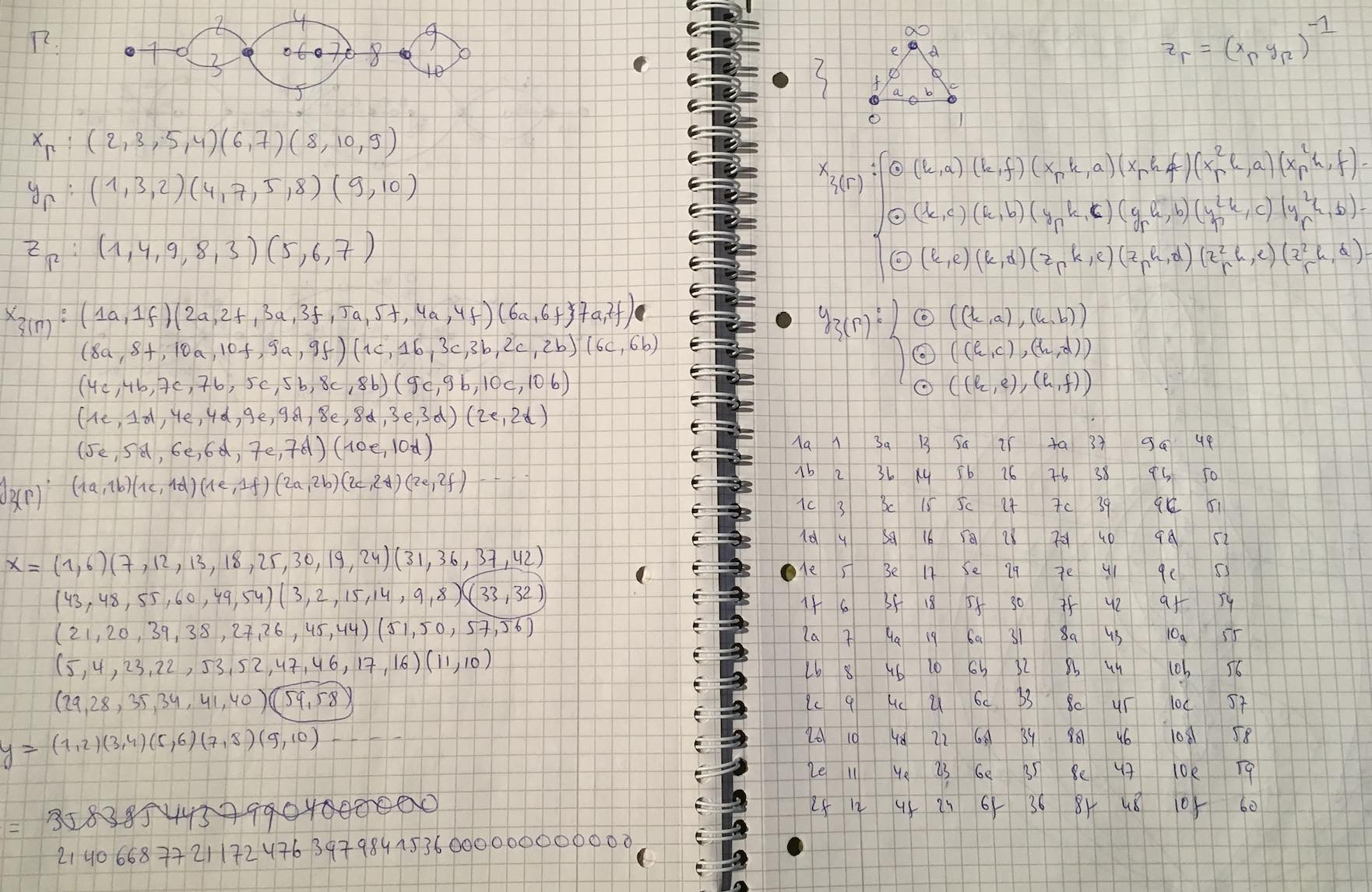Absolute geometry is the attempt to develop algebraic geometry over the elusive field with one element $\mathbb{F}_1$. The idea being that the set of all prime numbers is just too large for $\mathbf{Spec}(\mathbb{Z})$ to be a terminal object (as it is in the category of schemes).

So, one wants to view $\mathbf{Spec}(\mathbb{Z})$ as a geometric object over something ‘deeper’, the “absolute point” $\mathbf{Spec}(\mathbb{F}_1)$.

Starting with the paper by Bertrand Toen and Michel Vaquie, Under $\mathbf{Spec}(\mathbb{Z})$, topos theory entered this topic.

First there was the proposal by Jim Borger to view $\lambda$-rings as $\mathbb{F}_1$-algebras. More recently, Alain Connes and Katia Consani introduced the arithmetic site.

Now, there are lectures series on these two approaches, one by Yuri I. Manin, the other by Alain Connes.

.

Yuri I. Manin in Ghent

On Tuesday, February 3rd, Yuri I. Manin will give the inaugural lectures of the new $\mathbb{F}_1$-seminars at Ghent University, organised by Koen Thas.

Coffee will be served from 13.00 till 14.00 at the Department of Mathematics, Ghent University, Krijgslaan 281, Building S22 and from 14.00 till 16.30 there will be lectures in the Emmy Noether lecture room, Building S25:

14:00 – 14:25: Introduction (by K. Thas)
14:30 – 15:20: Lecture 1 (by Yu. I. Manin)
15:30 – 16:20: Lecture 2 (by Yu. I. Manin)

Recent work of Manin related to $\mathbb{F}_1$ includes:

Alain Connes on the Arithmetic Site

Until the beginning of march, Alain Connes will lecture every thursday afternoon from 14.00 till 17.30, in Salle 5 – Marcelin Berthelot at he College de France on The Arithmetic Site (hat tip Isar Stubbe).

Here’s a two minute excerpt, from a longer interview with Connes, on the arithmetic site, together with an attempt to provide subtitles:

——————————————————

(50.36)

And,in this example, we saw the wonderful notion of a topos, developed by Grothendieck.

It was sufficient for me to open SGA4, a book written at the beginning of the 60ties or the late fifties.

It was sufficient for me to open SGA4 to see that all the things that I needed were there, say, how to construct a cohomology on this site, how to develop things, how to see that the category of sheaves of Abelian groups is an Abelian category, having sufficient injective objects, and so on … all those things were there.

This is really remarkable, because what does it mean?

It means that the average mathematician says: “topos = a generalised topological space and I will never need to use such things. Well, there is the etale cohomology and I can use it to make sense of simply connected spaces and, bon, there’s the chrystaline cohomology, which is already a bit more complicated, but I will never need it, so I can safely ignore it.”

And (s)he puts the notion of a topos in a certain category of things which are generalisations of things, developed only to be generalisations…

But in fact, reality is completely different!

In our work with Katia Consani we saw not only that there is this epicyclic topos, but in fact, this epicyclic topos lies over a site, which we call the arithmetic site, which itself is of a delirious simplicity.

It relies only on the natural numbers, viewed multiplicatively.

That is, one takes a small category consisting of just one object, having this monoid as its endomorphisms, and one considers the corresponding topos.

This appears well … infantile, but nevertheless, this object conceils many wonderful things.

And we would have never discovered those things, if we hadn’t had the general notion of what a topos is, of what a point of a topos is, in terms of flat functors, etc. etc.

(52.27)

——————————————————-

I will try to report here on Manin’s lectures in Ghent. If someone is able to attend Connes’ lectures in Paris, I’d love to receive updates!

Almost three decades ago, Yuri Manin submitted the paper “New dimensions in geometry” to the 25th Arbeitstagung, Bonn 1984. It is published in its proceedings, Springer Lecture Notes in Mathematics 1111, 59-101 and there’s a review of the paper available online in the Bulletin of the AMS written by Daniel Burns.

In the introduction Manin makes some highly speculative but inspiring conjectures. He considers the ring

$$\mathbb{Z}[x_1,\ldots,x_m;\xi_1,\ldots,\xi_n]$$

where $\mathbb{Z}$ are the integers, the $\xi_i$ are the “odd” variables anti-commuting among themselves and commuting with the “even” variables $x_j$. To this ring, Manin wants to associate a geometric object of dimension $1+m+n$ where $1$ refers to the “arithmetic dimension”, $m$ to the ordinary geometric dimensions $(x_1,\ldots,x_m)$ and $n$ to the new “odd dimensions” represented by the coordinates $(\xi_1,\ldots,\xi_n)$. Manin writes :

“Before the advent of ringed spaces in the fifties it would have been difficult to say precisely what me mean when we speak about this geometric object. Nowadays we simply define it as an “affine superscheme”, an object of the category of topological spaces locally ringed by a sheaf of $\mathbb{Z}_2$-graded supercommutative rings.”

Here’s my own image (based on Mumford’s depiction of $\mathsf{Spec}(\mathbb{Z}[x])$) of what Manin calls the three-space-2000, whose plain $x$-axis is supplemented by the set of primes and by the “black arrow”, corresponding to the odd dimension.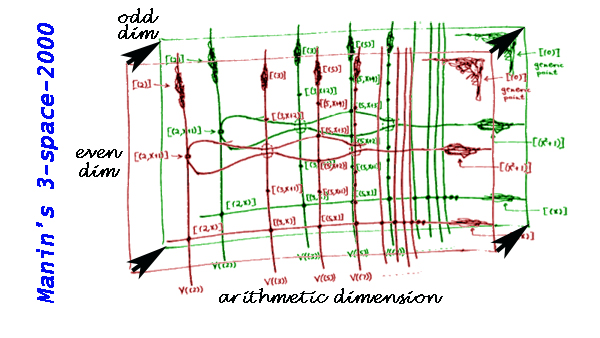Manin speculates : “The message of the picture is intended to be the following metaphysics underlying certain recent developments in geometry: all three types of geometric dimensions are on an equal footing”.

Probably, by the addition “2000” Manin meant that by the year 2000 we would as easily switch between these three types of dimensions as we were able to draw arithmetic schemes in the mid-80ties. Quod non.

Twelve years into the new millenium we are only able to decode fragments of this. We know that symmetric algebras and exterior algebras (that is the “even” versus the “odd” dimensions) are related by Koszul duality, and that the precise relationship between the arithmetic axis and the geometric axis is the holy grail of geometry over the field with one element.

For aficionados of $\mathbb{F}_1$ there’s this gem by Manin to contemplate :

“Does there exist a group, mixing the arithmetic dimension with the (even) geometric ones?”

Way back in 1984 Manin conjectured : “There is no such group naively, but a ‘category of representations of this group’ may well exist. There may exist also certain correspondence rings (or their representations) between $\mathsf{Spec}(\mathbb{Z})$ and $x$.”

Previously, we have recalled comparisons between approaches to define a geometry over the absolute point and art-historical movements, first those due to Yuri I. Manin, subsequently some extra ones due to Javier Lopez Pena and Oliver Lorscheid.

In these comparisons, the art trend appears to have been chosen more to illustrate a key feature of the approach or an appreciation of its importance, rather than giving a visual illustration of the varieties over $\mathbb{F}_1$ the approach proposes.

Some time ago, we’ve had a couple of posts trying to depict noncommutative varieties, first the illustrations used by Shahn Majid and Matilde Marcolli, and next my own mental picture of it.

In this post, we’ll try to do something similar for affine varieties over the absolute point. To simplify things drastically, I’ll divide the islands in the Lopez Pena-Lorscheid map of $\mathbb{F}_1$ land in two subsets : the former approaches (all but the $\Lambda$-schemes) and the current approach (the $\Lambda$-scheme approach due to James Borger).

The former approaches : Francis Bacon “The Pope” (1953)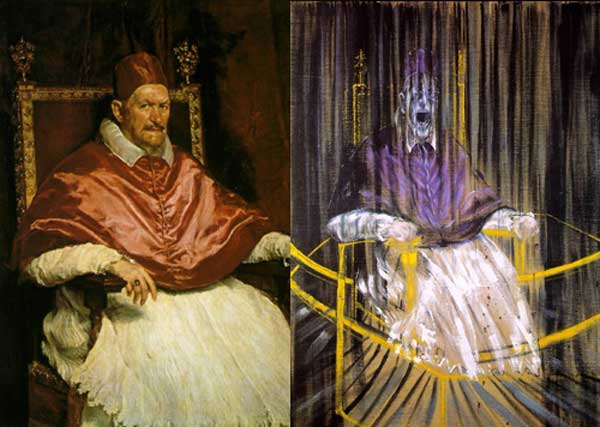The general consensus here was that in going from $\mathbb{Z}$ to $\mathbb{F}_1$ one looses the additive structure and retains only the multiplicative one. Hence, ‘commutative algebras’ over $\mathbb{F}_1$ are (commutative) monoids, and mimicking Grothendieck’s functor of points approach to algebraic geometry, a scheme over $\mathbb{F}_1$ would then correspond to a functor

$h_Z~:~\mathbf{monoids} \longrightarrow \mathbf{sets}$

Such functors are described largely by combinatorial data (see for example the recent blueprint-paper by Oliver Lorscheid), and, if the story would stop here, any Rothko painting could be used as illustration.

Most of the former approaches add something though (buzzwords include ‘Arakelov’, ‘completion at $\infty$’, ‘real place’ etc.) in order to connect the virtual geometric object over $\mathbb{F}_1$ with existing real, complex or integral schemes. For example, one can make the virtual object visible via an evaluation map $h_Z \rightarrow h_X$ which is a natural transformation, where $X$ is a complex variety with its usual functor of points $h_X$ and to connect both we associate to a monoid $M$ its complex monoid-algebra $\mathbb{C} M$. An integral scheme $Y$ can then be said to be ‘defined over $\mathbb{F}_1$’, if $h_Z$ becomes a subfunctor of its usual functor of points $h_Y$ (again, assigning to a monoid its integral monoid algebra $\mathbb{Z} M$) and $Y$ is the ‘best’ integral scheme approximation of the complex evaluation map.

To illustrate this, consider the painting Study after Velázquez’s Portrait of Pope Innocent X by Francis Bacon (right-hand painting above) which is a distorded version of the left-hand painting Portrait of Innocent X by Diego Velázquez.

Here, Velázquez’ painting plays the role of the complex variety which makes the combinatorial gadget $h_Z$ visible, and, Bacon’s painting depicts the integral scheme, build up from this combinatorial data, which approximates the evaluation map best.

All of the former approaches more or less give the same very small list of integral schemes defined over $\mathbb{F}_1$, none of them motivically interesting.

The current approach : Jackson Pollock “No. 8” (1949)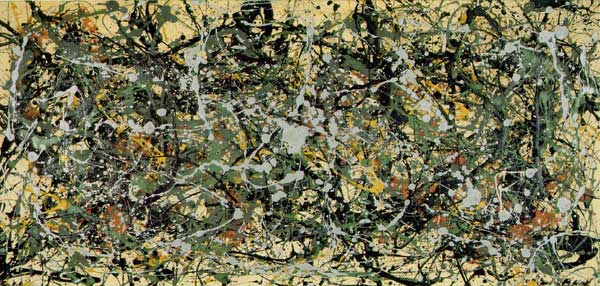An entirely different approach was proposed by James Borger in $\Lambda$-rings and the field with one element. He proposes another definition for commutative $\mathbb{F}_1$-algebras, namely $\lambda$-rings (in the sense of Grothendieck’s Riemann-Roch) and he argues that the $\lambda$-ring structure (which amounts in the sensible cases to a family of endomorphisms of the integral ring lifting the Frobenius morphisms) can be viewed as descent data from $\mathbb{Z}$ to $\mathbb{F}_1$.

The list of integral schemes of finite type with a $\lambda$-structure coincides roughly with the list of integral schemes defined over $\mathbb{F}_1$ in the other approaches, but Borger’s theory really shines in that it proposes long sought for mystery-objects such as $\mathbf{spec}(\mathbb{Z}) \times_{\mathbf{spec}(\mathbb{F}_1)} \mathbf{spec}(\mathbb{Z})$. If one accepts Borger’s premise, then this object should be the geometric object corresponding to the Witt-ring $W(\mathbb{Z})$. Recall that the role of Witt-rings in $\mathbb{F}_1$-geometry was anticipated by Manin in Cyclotomy and analytic geometry over $\mathbb{F}_1$.

But, Witt-rings and their associated Witt-spaces are huge objects, so one needs to extend arithmetic geometry drastically to include such ‘integral schemes of infinite type’. Borger has made a couple of steps in this direction in The basic geometry of Witt vectors, II: Spaces.

To depict these new infinite dimensional geometric objects I’ve chosen for Jackson Pollock‘s painting No. 8. It is no coincidence that Pollock-paintings also appeared in the depiction of noncommutative spaces. In fact, Matilde Marcolli has made the connection between $\lambda$-rings and noncommutative geometry in Cyclotomy and endomotives by showing that the Bost-Connes endomotives are universal for $\lambda$-rings.

One of the more surprising analogies around is that prime numbers can be viewed as knots in the 3-sphere $S^3$. The motivation behind it is that the (etale) fundamental group of $\pmb{spec}(\mathbb{Z}/(p))$ is equal to (the completion) of the fundamental group of a circle $S^1$ and that the embedding

$\pmb{spec}(\mathbb{Z}/(p)) \subset \pmb{spec}(\mathbb{Z})$

embeds this circle as a knot in a 3-dimensional simply connected manifold which, after Perelman, has to be $S^3$. For more see the what is the knot associated to a prime?-post.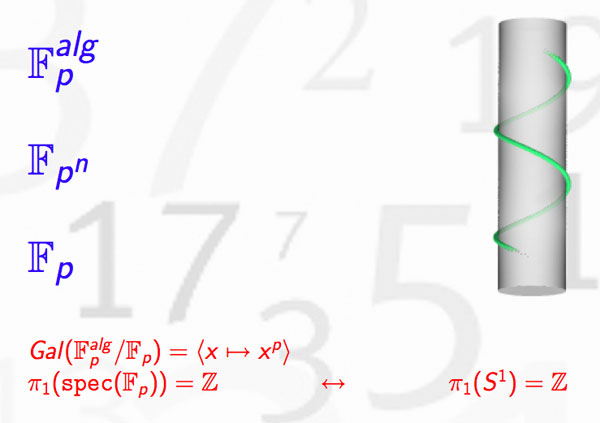In recent months new evidence has come to light allowing us to settle the genesis of this marvelous idea.

1. The former consensusUntil now, the generally accepted view (see for example the ‘Mazur-dictionary-post’ or Morishita’s expository paper) was that the analogy between knots and primes was first pointed out by Barry Mazur in the middle of the 1960’s when preparing for his lectures at the Summer Conference on Algebraic Geometry, at Bowdoin, in 1966. The lecture notes where later published in 1973 in the Annales of the ENS as ‘Notes on etale cohomology of number fields’.

For further use in this series of posts, please note the acknowledgement at the bottom of the first page, reproduced below : “It gives me pleasure to thank J.-P. Serre for his vigorous editing and his suggestions and corrections, which led to this revised version.”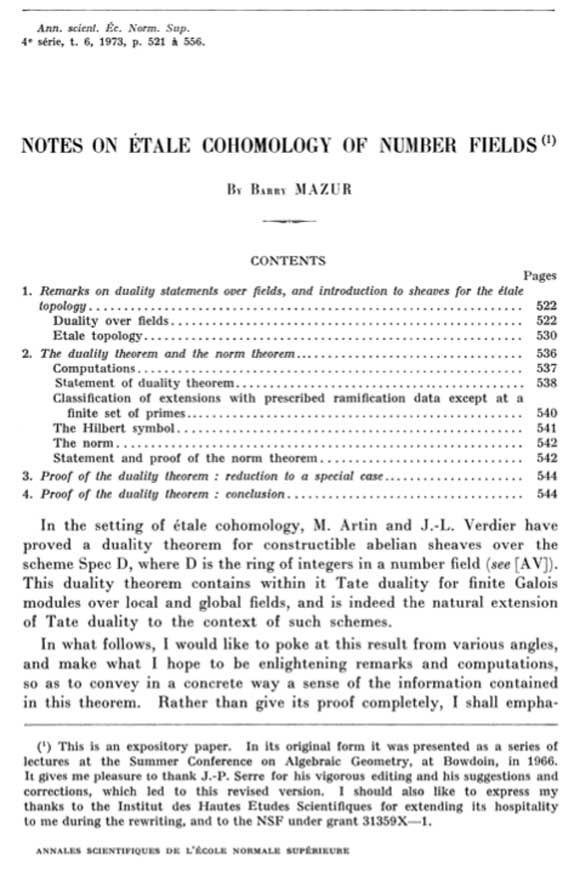Independently, Yuri I. Manin spotted the same analogy at around the same time. However, this point of view was quickly forgotten in favor of the more classical one of viewing number fields as analogous to algebraic function fields of one variable. Subsequently, in the mid 1990’s Mikhail Kapranov and Alexander Reznikov took up the analogy between number fields and 3-manifolds again, and called the resulting study arithmetic topology.

2. The new evidence

On december 13th 2010, David Feldman posted a MathOverflow-question Mazur’s unpublished manuscript on primes and knots?. He wrote : “The story of the analogy between knots and primes, which now has a literature, started with an unpublished note by Barry Mazur. I’m not absolutely sure this is the one I mean, but in his paper, Analogies between group actions on 3-manifolds and number fields, Adam Sikora cites B. Mazur, Remarks on the Alexander polynomial, unpublished notes.

Two months later, on february 15th David Feldman suddenly found the missing preprint in his mail-box and made it available. The preprint is now also available from Barry Mazur’s website. Mazur adds the following comment :

“In 1963 or 1964 I wrote an article Remarks on the Alexander Polynomial [PDF] about the analogy between knots in the three-dimensional sphere and prime numbers (and, correspondingly, the relationship between the Alexander polynomial and Iwasawa Theory). I distributed some copies of my article but never published it, and I misplaced my own copy. In subsequent years I have had many requests for my article and would often try to search through my files to find it, but never did. A few weeks ago Minh-Tri Do asked me for my article, and when I said I had none, he very kindly went on the web and magically found a scanned copy of it. I’m extremely grateful to Minh-Tri Do for his efforts (and many thanks, too, to David Feldman who provided the lead).”The opening paragraph of this unpublished preprint contains a major surprise!

Mazur points to David Mumford as the originator of the ‘primes-are-knots’ idea : “Mumford has suggested a most elegant model as a geometric interpretation of the above situation : $\pmb{spec}(\mathbb{Z}/p\mathbb{Z})$ is like a one-dimensional knot in $\pmb{spec}(\mathbb{Z})$ which is like a simply connected three-manifold.”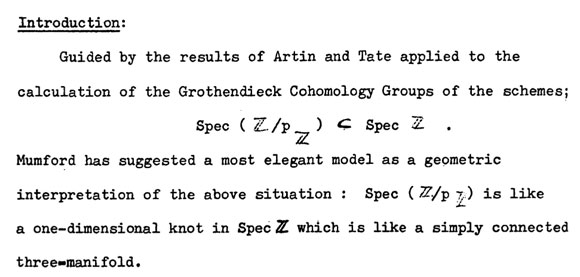In a later post we will show that one can even pinpoint the time and place when and where this analogy was first dreamed-up to within a few days and a couple of miles.

For the impatient among you, have a sneak preview of the cradle of birth of the primes=knots idea…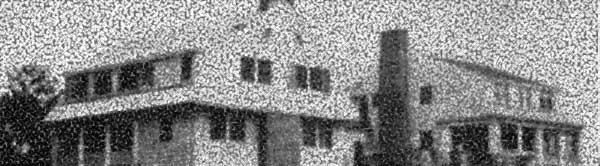Last time we did recall Manin’s comparisons between some approaches to geometry over the absolute point $\pmb{spec}(\mathbb{F}_1)$ and trends in the history of art.

In the comments to that post, Javier Lopez-Pena wrote that he and Oliver Lorscheid briefly contemplated the idea of extending Manin’s artsy-dictionary to all approaches they did draw on their Map of $\mathbb{F}_1$-land.

So this time, we will include here Javier’s and Oliver’s insights on the colored pieces below in their map : CC=Connes-Consani, Generalized torified schemes=Lopez Pena-Lorscheid, Generalized schemes with 0=Durov and, this time, $\Lambda$=Manin-Marcolli.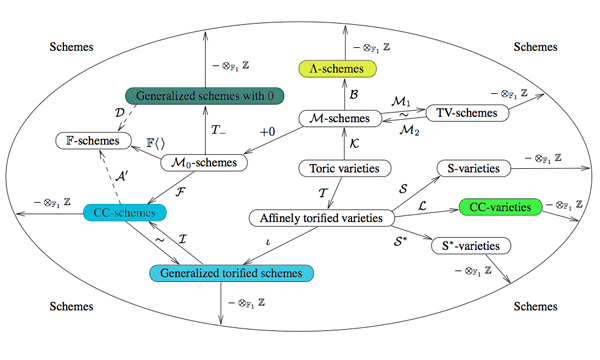Durov : romanticismIn his 568 page long Ph.D. thesis New Approach to Arakelov Geometry Nikolai Durov introduces a vast generalization of classical algebraic geometry in which both Arakelov geometry and a more exotic geometry over $\mathbb{F}_1$ fit naturally. Because there were great hopes and expectations it would lead to a big extension of algebraic geometry, Javier and Oliver associate this approach to romantism. From wikipedia : “The modern sense of a romantic character may be expressed in Byronic ideals of a gifted, perhaps misunderstood loner, creatively following the dictates of his inspiration rather than the standard ways of contemporary society.”

Manin and Marcolli : impressionismYuri I. Manin in Cyclotomy and analytic geometry over $\mathbb{F}_1$ and Matilde Marcolli in Cyclotomy and endomotives develop a theory of analytic geometry over $\mathbb{F}_1$ based on analytic functions ‘leaking out of roots of unity’. Javier and Oliver depict such functions as ‘thin, but visible brush strokes at roots of 1’ and therefore associate this approach to impressionism. Frow wikipedia : ‘Characteristics of Impressionist paintings include: relatively small, thin, yet visible brush strokes; open composition; emphasis on accurate depiction of light in its changing qualities (often accentuating the effects of the passage of time); common, ordinary subject matter; the inclusion of movement as a crucial element of human perception and experience; and unusual visual angles.’

Connes and Consani : cubismIn On the notion of geometry over $\mathbb{F}_1$ Alain Connes and Katia Consani develop their extension of Soule’s approach. A while ago I’ve done a couple of posts on this here, here and here. Javier and Oliver associate this approach to cubism (a.o. Pablo Picasso and Georges Braque) because of the weird juxtapositions of the simple monoidal pieces in this approach.

Lopez-Pena and Lorscheid : deconstructivismTorified varieties and schemes were introduced by Javier Lopez-Pena and Oliver Lorscheid in Torified varieties and their geometries over $\mathbb{F}_1$ to get lots of examples of varieties over the absolute point in the sense of both Soule and Connes-Consani. Because they were fragmenting schemes into their “fundamental pieces” they associate their approach to deconstructivism.

Another time I’ll sketch my own arty-farty take on all this.In his paper Cyclotomy and analytic geometry over $\mathbb{F}_1$ Yuri I. Manin sketches and compares four approaches to the definition of a geometry over $\mathbb{F}_1$, the elusive field with one element.

He writes : “Preparing a colloquium talk in Paris, I have succumbed to the temptation to associate them with some dominant trends in the history of art.”

Remember that the search for the absolute point $\pmb{spec}(\mathbb{F}_1)$ originates from the observation that $\pmb{spec}(\mathbb{Z})$, the set of all prime numbers together with $0$, is too large to serve as the terminal object in Grothendieck’s theory of commutative schemes. The last couple of years have seen a booming industry of proposals, to the extent that Javier Lopez Pena and Oliver Lorscheid decided they had to draw a map of $\mathbb{F}_1$-land.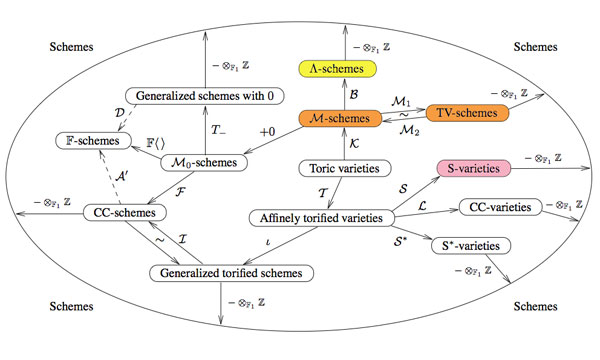Manin only discusses the colored proposals (TV=Toen-Vaquie, M=Deitmar, S=Soule and $\Lambda$=Borger) and compares them to these art-history trends.

Toen and Vaquie : Abstract ExpressionismIn Under $\pmb{spec}(\mathbb{Z})$ Bertrand Toen and Michel Vaquie argue that geometry over $\mathbb{F}_1$ is a special case of algebraic geometry over a symmetric monoidal category, taking the simplest example namely sets and direct products. Probably because of its richness and abstract nature, Manin associates this approach to Abstract Expressionism (a.o. Karel Appel, Jackson Pollock, Mark Rothko, Willem de Kooning).

Deitmar : MinimalismBecause monoids are the ‘commutative algebras’ in sets with direct products, an equivalent proposal is that of Anton Deitmar in Schemes over $\mathbb{F}_1$ in which the basic affine building blocks are spectra of monoids, topological spaces whose points are submonoids satisfying a primeness property. Because Deitmar himself calls this approach a ‘minimalistic’ one it is only natural to associate to it Minimalism where the work is stripped down to its most fundamental features. Prominent artists associated with this movement include Donald Judd, John McLaughlin, Agnes Martin, Dan Flavin, Robert Morris, Anne Truitt, and Frank Stella.

Soule : Critical Realismin Les varietes sur le corps a un element Christophe Soule defines varieties over $\mathbb{F}_1$ to be specific schemes $X$ over $\mathbb{Z}$ together with a form of ‘descent data’ as well as an additional $\mathbb{C}$-algebra, morally the algebra of functions on the real place. Because of this Manin associates to it Critical Realism in philosophy. There are also ‘realism’ movements in art such as American Realism (o.a. Edward Hopper and John Sloan).

Borger : FuturismJames Borger’s paper Lambda-rings and the field with one element offers a totally new conception of the descent data from $\mathbb{Z}$ to $\mathbb{F}_1$, namely that of a $\lambda$-ring in the sense of Grothendieck. Because Manin expects this approach to lead to progress in the field, he connects it to Futurism, an artistic and social movement that originated in Italy in the early 20th century.